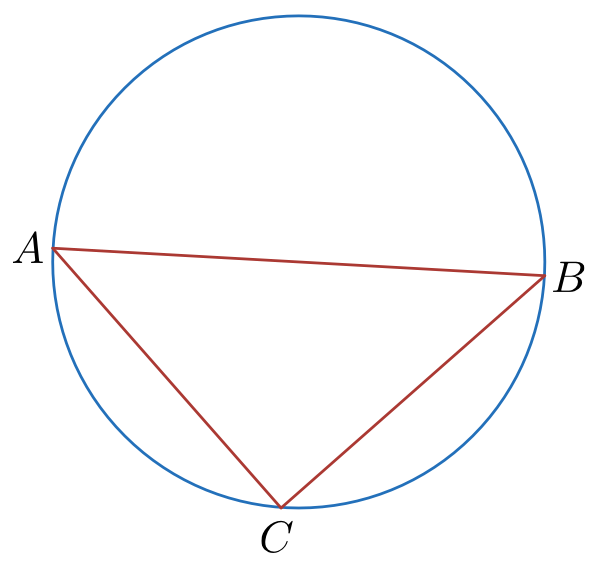# Inscribed Triangle - Easy Geometry

Geometry Level 2A circle has an area equal to 25$\pi$ $cm^2$ . Its diameter AB coincides with one of the sides of triangle ACB in which the vertex C lies on the circle. If the triangle has an area equal to 11 $cm^2$, find its perimeter.

×